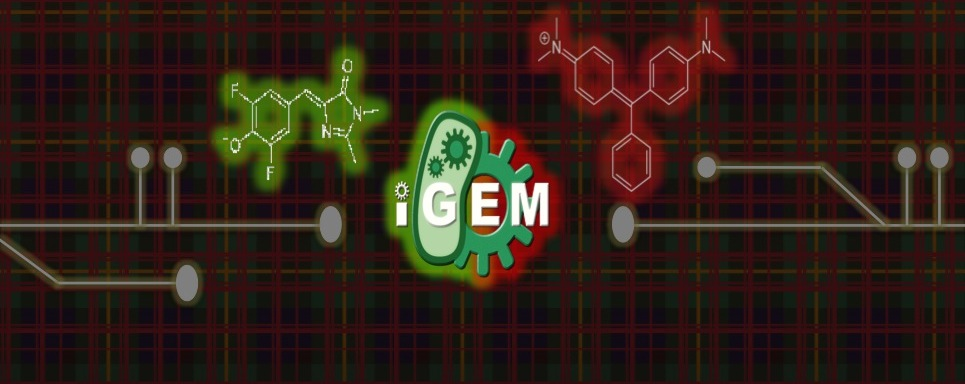# Team:Carnegie Mellon/Mod-Matlab# Inputs

The inputs to the model are the measurement tables of concentration of dye vs. time. Optional inputs to the model include an in vitro measurement of saturation of the dye, and measurements of the fluorescence of the dye with mRNA and protein synthesis turned off. The first optional measurement can be used to compare the in vitro fluorescence saturation levels with the in vivo fluorescence saturation levels in order to give a scaling factor for the all the measurements in the input. Estimations can be used in place of these to simplify the number of inputs. The second optional measurement can be used to determine the degradation rates of mRNA and protein.

# Walkthrough

Fluoro2.m

This function is the function that is called to run the entire program. In addition, it takes the mRNA titration tables (modeldata) and converts it into fluorescent mRNA concentrations. It then passes the degradation data to the degradation functions, Degradation.m and DegradationP.m to return alpha2 and beta2, the degradation coefficients.

This function takes in mRNA fluorescence data with mRNA synthesis turned off. This makes determining degradation rates easier, as all the change (as we will define degradation) in the mRNA concentration will be due to degradation. The function takes the data and fits a curve to the data, in the process calculating the degradation rate.This function has a similar role to Degradation.m. This function takes in protein fluorescence data with protein synthesis turned off. This function, similarly to Degradation.m, takes the data and fits a curve to the data.

The degradation functions return alpha2 and beta2 to Fluoro2.m. Fluoro2.m then calls ProteinFunctions.m to convert the protein titration data to fluorescent protein concentrations.

ProteinFunctions.m

This function does the same thing as Fluoro2.m with the mRNA titration data. It returns fluorescent protein concentrations over time.

Fluoro2.m takes the fluorescent protein and fluorescent mRNA concentrations and passes them to FluoroLR.m and FluoroLP.m to convert to total protein and total mRNA concentrations.

FluoroLR.m

This function takes in fluorescent mRNA concentrations and converts it to mRNA concentrations using first-order chemical reactions. One dye molecule will bond to one mRNA molecule, creating an mRNA-dye complex. This leads to a rather simple conversion using the known dye concentration.

Where KDR is the dissociation constant, [Rf] is the fluorescent mRNA concentration, [R] is the mRNA concentration, and [DR] is the dye concentration.

FluoroLP.m

This function has a similar role to FluoroLR.m, except using fluorescent protein concentrations and converting it to protein concentration.

mRNAexpress.m

Fluoro2.m then takes the total mRNA concentrations passed by FluoroLR.m and passes it to mRNAexpress.m, which calculates the transcriptional efficiency. This is done via the differential equationto which the solution iswhere [R] is mRNA concentration, Ts is transcriptional efficiency, [D] is DNA concentration, and alpha is the mRNA degradation coefficient.

proteinexpress.m

Fluoro2.m takes the transcriptional efficiency from mRNAexpress.m, total protein concentrations from FluoroLP.m, and alpha2 and beta2 from Degradation.m and DegradationP.m, and passes them to proteinexpress.m. proteinexpress.m computes the translational efficiency using the differential equationto which the solution iswhere Tl is translational efficiency, beta is the protein degradation coefficient, and [P] is the protein concentration.

PoPS.m

Fluoro2.m passes to the final function alpha2 and beta2 from Degradation.m and DegradationP.m, total protein concentration from FluoroLP.m, and translational efficiency from proteinexpress.m. PoPS.m calculates the approximate polymerase per second using the equationwhere n is the approximate number of the promoters of interest in a cell (i.e. plasmid copy).

# Outputs

The model outputs polymerase per second, although transcriptional efficiency and translational efficiency are also important factors in the model. Derivations of these equations can be found on the derivations page.